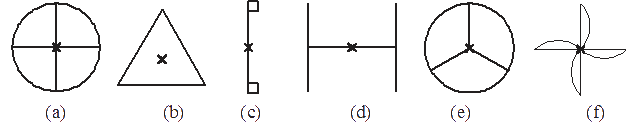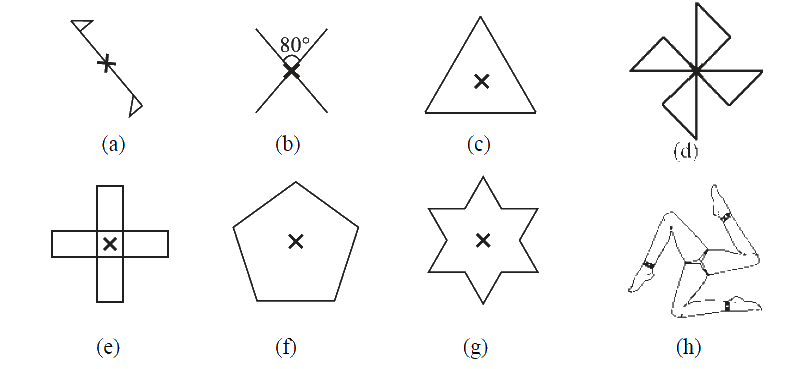# Exercise 14.2 Symmetry -NCERT Solutions Class 7

Go back to  'Symmetry'

## Chapter 14 Ex.14.2 Question 1

Which of the following figures have rotational symmetry of order more than $$1$$:### Solution

What is known?

Figures

What is unknown?

The figures have rotational symmetry of order more than $$1$$.

Reasoning:

To solve this question, remember the concept of rotational symmetry. Rotational symmetry is when an object is rotated around a center point (turned) a number of degrees and the object appears the same. The order of symmetry is the number of positions the object looks the same in a $$360^\circ$$ rotation.

Steps:

Rotational symmetry of order more than one are: (a), (b), (c), (d), (e) and (f)  because in these figures, in a complete turn more than one number of times an object looks exactly the same.

## Chapter 14 Ex.14.2 Question 2

Give the order of rotational symmetry for each figure:### Solution

What is known?

Figures.

What is unknown?

The order of rotational symmetry

Reasoning:

To solve this question, remember the concept of rotational symmetry. Rotational symmetry is when an object is rotated around a centre point (turned) a number of degrees and the object appears the same. The order of symmetry is the number of positions the object looks the same in a $$360^\circ$$ rotation.

Steps:

 Figure Order of Rotational Symmetry a 2 b 2 c 3 d 4 e 4 f 5 g 6 h 3

Related Sections
Related Sections
Instant doubt clearing with Cuemath Advanced Math Program